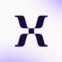Formulas: Calculating with numeric values - Mixpanel
Blog Post

# Formulas: Calculating with numeric values

Last edited: Sep 13, 2022 Published: Jan 16, 2015Mixpanel Team

When it comes to Mixpanel products, we’re all about constantly making improvements that our users have asked for. Regarding the formulas report, one thing we’ve had numerous requests for is the ability to add numeric values into a formula. Well Mixpanellers, ask and ye shall receive:

The most basic and useful application of this is a formula where you would like to see percentage values rather than decimals. In our case, let’s look at a common event and take the number of mobile occurrences divided by total occurrences:

As you can see, the x-axis values are calculated as decimals between 0 and 1. In order to transform the values in this chart into percentages, all we have to do is multiply the numerator by 100:

And there you have it, a simple addition to formulas that greatly increases their flexibility. For more use cases and ideas, get in touch with our extremely knowledgeable support team – support@mixpanel.com.

Get the latest from Mixpanel
This field is required.